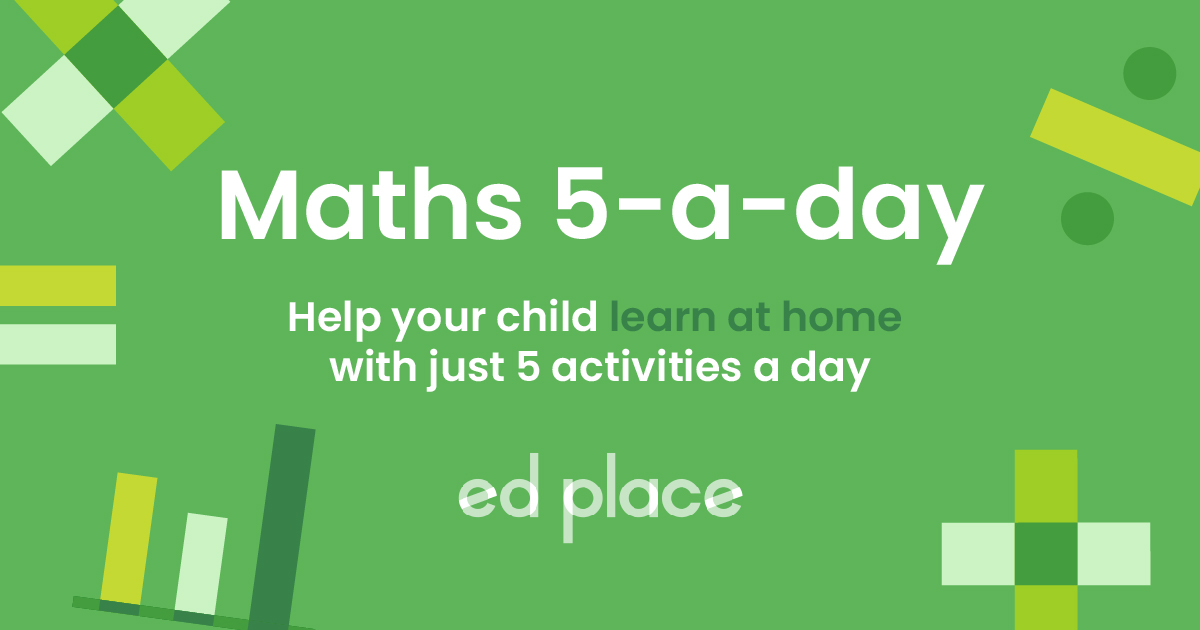# EdPlace's Year 5 and 6 home learning maths lesson: Dividing Fractions

Looking for short lessons to keep your child engaged and learning? Our experienced team of teachers have created English, maths and science lessons for the home, so your child can learn no matter where they are.  And, as all activities are self-marked, you really can encourage your child to be an independent learner.

Get them started on the lesson below and then jump into our teacher-created activities to practice what they've learnt. We've recommended five to ensure they feel secure in their knowledge - 5-a-day helps keeps the learning loss at bay (or so we think!).

Are they keen to start practising straight away? Head to the bottom of the page to find the activities.

Now...onto the lesson!

Upper Key Stage 2 Statutory Requirements for Maths
Year 6 students should be taught to divide proper fractions by whole numbers.

## Learning Objectives:

We're confident that if you follow this step-by-step approach together, your child will be able to:

1) Understand how to divide any fraction by a whole number.

2) Apply this to independent work.

3) Explain to you how they completed their work, if they've really cracked it!

## How on earth do we divide fractions?!

What is 1/6 ÷ 3?

If we remember the good old acronym ‘KFC’, it becomes quite straightforward!

This article will teach you how to divide fractions by a whole number.

## Step 1 - Key Terminology

Divide – share something equally.

Simplify – reduce a fraction to its simplest form, by dividing the numerator (number on top) and the denominator (number on the bottom) by the highest common factor (the largest number than can go into both the numerator and the denominator).

## Step 2 - Prior Understanding

Your child will need to be able to simplify fractions, by finding the highest common factor and dividing the numerator and denominator by this factor.

Example: 5/35 the highest common factor is 5 (both numbers can be divided by 5)

1/7 is 5/35 in its simplest form.

Your child also needs to understand that any whole number can be written as a fraction over 1, e.g. 5 is the same as 5/1 or 12 is the same as 12/1.

They need to know how to multiply fractions – you just multiply the numerators together, and multiply the denominators together, so 2/9 x 3/5 = 6/45.

## Step 3 - Introducing a New Skill

The main thing you need to remember is KFC: Keep, Flip, Change

Keep the first fraction the same

Flip the second fraction round

Change the operation (from ÷ to x)

Examples:

1. 1/4 ÷ 2   =   1/4 ÷ 2/1   =  1/4 x 1/2 = 1/8

K – keep first fraction the same ( ¼ )

F – flip second fraction (it changes from 2/1 to 1/2)

C – change from ÷ to x

1. 3/4 ÷ 6   =   3/4 ÷ 6/1   =   3/4 x 1/6 = 3/24,  3 and 24 can both be divided by 3 so the answer is 1/8.

You might also notice that a faster way would be to ignore the numerator (keep it the same) and multiply the denominator by the whole number:

1. 2/7 ÷ 2 = 2/14   Don’t forget to simplify it!  =  1/7.

Keep the numerator the same (2), then do 7 x 2 (denominator x whole number)

## Step 4 - Putting it into Practice

Now have a go at these examples together:

A) 1/6 ÷ 3

B) 1/7 ÷ 5

C) 3/5 ÷ 3

D) 2/9 ÷ 4

Challenge questions: (remember KFC)

E) 3/4 ÷ 1/2

F) 7/9 ÷ 3/4

## Step 5 - Give it a go...

Why not test your child's understanding and see if they can tackle these activities?

All activities are created by teachers and automatically marked. Plus, with an EdPlace subscription, we can automatically progress your child at a level that's right for them. Sending you progress reports along the way so you can track and measure progress, together - brilliant!

Main activities: (make sure you reduce them to their simplest form)

Extension activities: use your division knowledge to convert these fractions into decimals – read the instructions carefully so you know what to do

A) 1/18

B) 1/35

C) 3/15 = 1/5

D) 2/36 = 1/18

E) 3/4 x 2/1 = 6/4 = 12/6 = 11/3

F) 7/9 x 4/3 = 28/27 = 11/27

Keep going! Looking for more activities, different subjects or year groups?

Click the button below to view the EdPlace English, maths, science and 11+ activity library